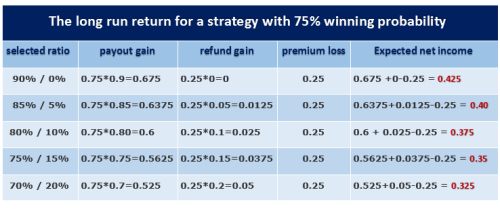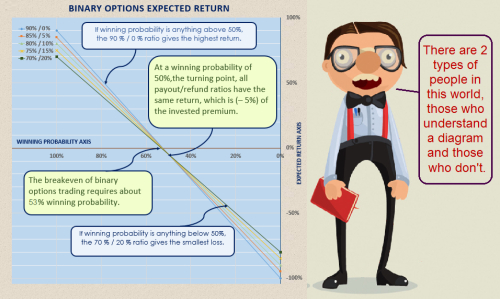Dukascopy gives binary options traders, in every trade, the right to select between predefined sets of Payout/Refund combinations. Combinations alternatives are : 90%/0% , 85%/5% , 80%/10% , 75%/15% , and 70%/20%.
For some traders it is puzzling. Traders may ask: which ratio is better to choose? Is it just a matter of personal preference and risk tolerance? Do all ratios have the same profit potential in the long run? If no, which ratio is more profitable? In this article we will try to answer these questions and help binary options traders to make a clear and intelligible choice.
P.S. If at any time you feel that you don't like the math details, you can directly jump to the article conclusion.What are Dukascopy payout / refund ratios?

Binary options payout ratio (90%, 85%, 80%, 75%, and 70%) refers to the percentage of your initial investment amount you will win if your prediction turns out to be correct and your option expires "in the money".

Whereas the binary options refund ratio, also known as "Return on loss" (0%, 5%, 10%, 15%, and 20%) refers to the percentage of your initial investment that you recover even if you traded in the wrong direction. You can get this partial refund only if your binary option expires “out of the money”. In such condition, you don’t lose your entire invested cash but get back a percentage of it that corresponds to the refund rate.

In every trade, clients are to choose one payout / refund ratio that best correspond to their personal risk tolerance. This means trader may select a lower payout percentage in exchange for a refund of part of the premium in case the contract expires with a loss.

For example, if your trade amount (the premium) is 100\$ and you select the 90% / 0% payout / refund ratio, you will get 90\$ profit if your option expires in the money, but if your option expires out of the money you will lose the entire 100\$ without any refund. Whereas by selecting the 70%/20% payout / refund ratio you will get only 70\$ profit if your option expires in the money, but in case your option expires out of the money you will recover 20\$ of the 100\$ you lost.

Payout / Refund ratios and the winning probability

If we know in advance and for sure that our trade(s) is profitable (winning probability is 100%), we will choose the 90%/0% ratio which will pay us the maximum profit, whereas if we know for sure that our trade(s) will lose (winning probability is 0%) then the 70%/20% ratio can give us the maximum refund, but we will not enter this surely losing trade(s) from the beginning. So it is obvious that choosing the most profitable payout / refund ratio is related to the winning probability.

Knowing that, two questions may arise: for winning probabilities that range between 0% and 100 (e.g., 75%), what is the most profitable payout / refund ratio? and in first place how do we know our winning probability in advance?

To know the winning probability of our future trade(s), we don't have any better mean than to analyze our past trading record and assume that future trades will have the same winning probability as past trades. We get the past trading record from back testing our trading system or from trading our system previously either on a demo or live account. To calculate the winning probability, we divide the number of positive (profitable) trades by the total number of trades (positive and negative). This number is better as it gets closer to one. Any number above 0.60 is good.
For example, if we analyzed the last 80 trades for a system and found that 60 trades ended in the money and were profitable, winning probability = 60/80=0.75 or 75%.

The most profitable payout / refund ratio for a particular winning probability

Supposing our win probability is 75%, we can calculate the expected net return for every payout / refund ratio. The best ratio is the one that gives the heist expected net return.

The net expected return = the expected payout gain + the expected refund gain – the expected premium loss
the expected payout gain= winning probability * payout ratio
the expected refund gain = losing probability * refund ratio ... where losing probability = 1- winning probability
the expected premium loss = losing probability *1Now it is clear that the first alternative (90% / 0%) is the best because it gives expected return 42.5% of the traded premium which is the highest expected return.

The most profitable payout / refund ratio: The overall rule
We will examine every payout / refund ratio return at several winning probabilities ratios to save you from calculating them yourself and to get the overall rule.ِA strategy with 60% winning probability have an expected long run return of 14% of the capital traded if the 90%/0% ratio was selected. This return declines to 13%, 12%, 11% or 10% if another payout / refund ratios was selected.
For winning probabilities above 50%, the 90 % / 0% ratio gives the highest return. Whereas for winning probabilities below 50%, the 70 % / 20% ratio gives the smallest loss. At a winning probability of 50% all payout / refund ratios have the same return which is (– 0.05%) of the invested premium. Better payout / refund ratios for winning probabilities above 50% become worse for winning probabilities below 50%. So the 50% ratio is actually acting like a turning point.

Same table can be drawn as a diagram to easily see the whole pictureconclusion

• With Dukascopy conditions, binary options trading break-even requires about 53% winning probability. This result can be affirmed easily using a well-known formula but that is beyond the scope of this article.
• A binary options gambler with 50% winning probability can choose any payout / refund ratio and he will end up losing 5% of his traded capital on the long run. This result assumes that the gambler uses a sound money management method, otherwise he might commit a suicide and lose all his capital in one single trade. An important note here is that the gambler doesn't even have the 50% winning probability. That is because the instrument spread works against him and this may have a great effect especially in small expiration times like 1 minuet. So the gambler has actually a winning probability less than 50% .He can lower his losses by selecting the 70%/20% ratio with the highest refund, but he will end up losing anyway.
• The serious binary options trader with a winning probability of 60% at least is advised to select the 90%/0% ratio to get the highest return. This payout / refund ratio is the best choice for any winning probability above 50 %.
• The 85%/5%, 80%/10%, and the 75%/15% payout / refund ratios don't maximize the income at any winning probability level.
I Hope that you are now able to select your payout / refund ratio more intelligently knowing the profit potential of each ratio.Translate to English Show original